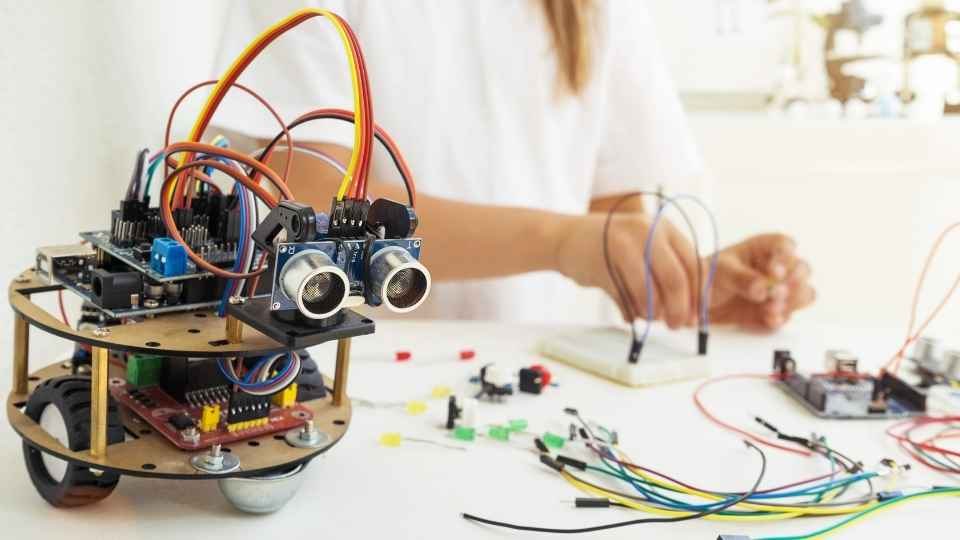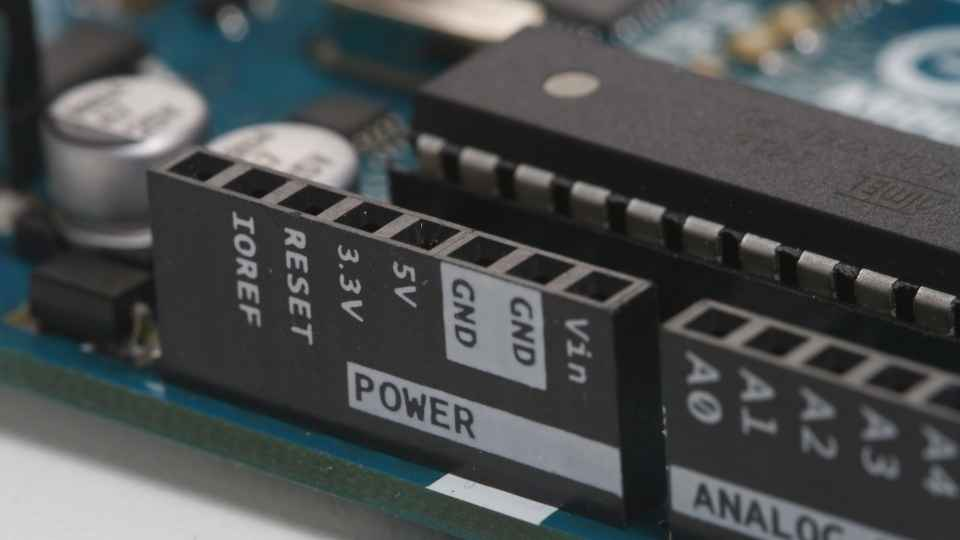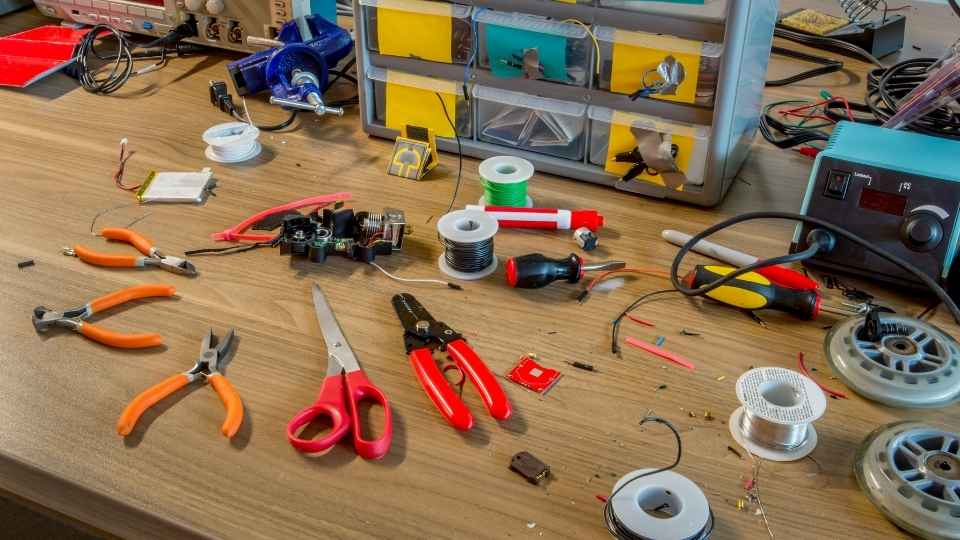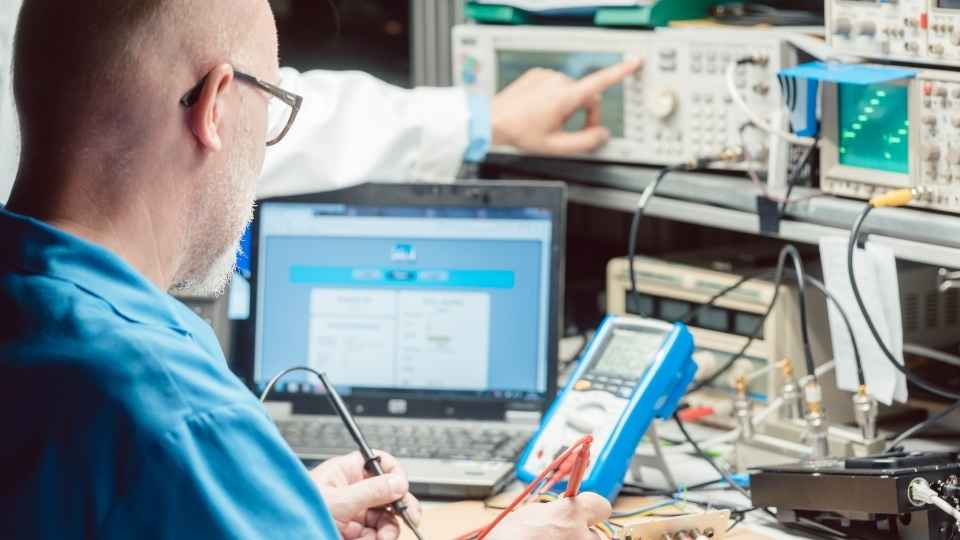# Digital Circuits: How Do Logic Gates and Binary Arithmetic Define Digital Worlds?Digital circuits are the lifeblood of our modern technological infrastructure, operating like an intricate web of interconnected neurons. Just as a symphony conductor orchestrates countless instruments to produce harmonious melodies, logic gates and binary arithmetic meticulously define the digital worlds we rely on.

In this article, we will delve into the technical intricacies of these foundational components, exploring their role in shaping digital systems and unveiling the power they hold in transforming bits and bytes into meaningful information.

Prepare to embark on a journey through the fascinating realm of digital circuits where logic reigns supreme.

## Key Takeaways

• Logic gates are the basic building blocks of digital systems and manipulate binary signals.
• Binary arithmetic involves logical operations like addition, subtraction, multiplication, and division using only 0 and 1.
• Flip-flops are fundamental for storing information in digital circuits until a triggering event occurs.
• Multiplexers enable the selection and routing of multiple input signals to a single output, reducing the need for physical components.

## The Role of Logic Gates in Digital Circuits

Logic gates play a fundamental role in digital circuits by manipulating binary signals to perform logical operations and create complex functions. These electronic devices are the building blocks of digital systems, allowing for the processing and manipulation of information in a precise and controlled manner.

There are several types of logic gates, including AND, OR, NOT, XOR, NAND, and NOR gates. Each gate has its own unique function and behavior based on Boolean algebra principles.

AND gates produce an output signal only when both input signals are high (logic 1), while OR gates produce an output signal when either or both input signals are high. NOT gates invert the input signal to its complement. XOR gates produce a high output when the number of high inputs is odd. NAND and NOR gates combine multiple logic operations into a single device.

By combining different logic gates in various configurations, complex logical functions can be achieved within digital circuits. These functions form the basis for tasks such as arithmetic calculations, data storage, communication protocols, and decision-making processes within computers and other digital devices.The versatility and flexibility provided by logic gates allow for the creation of sophisticated systems that enable individuals to exercise their freedom in designing innovative solutions. This freedom extends to engineers who have the ability to manipulate these logic gate arrangements to suit specific requirements or challenges they may encounter during circuit design.

## Understanding Binary Arithmetic and Its Application in Digital Worlds

By comprehending the principles of binary mathematics, one can gain a deeper understanding of how numerical operations are performed in the realm of digital systems. Binary arithmetic is based on the use of only two digits, 0 and 1, which correspond to off and on states in digital circuits. These digits are manipulated using logical operations such as addition, subtraction, multiplication, and division.

In binary addition, for example, each digit is added from right to left with consideration given to carry-over values. This allows for efficient manipulation of data in digital systems since all calculations can be reduced to simple binary operations.

Understanding binary arithmetic is crucial for designing and analyzing digital circuits as it forms the foundation for more complex computations and algorithms.

In the next section, we will explore the functionality of flip-flops in digital systems and how they contribute to storing and manipulating data within these circuits.

## Exploring the Functionality of Flip-Flops in Digital Systems

To comprehend the inner workings of digital systems, it is essential to delve into the functionality of flip-flops and how they contribute to the storage and manipulation of data.

Flip-flops are fundamental building blocks in digital circuits that can store a single bit of information. They are capable of maintaining their state until a triggering event occurs, making them vital for storing data in sequential logic circuits.One commonly used type of flip-flop is the D-type flip-flop, which has two inputs: a data input (D) and a clock input (CLK). When the clock signal transitions from low to high, the value at the data input is transferred to the output. This enables sequential circuits to hold and manipulate information over time, allowing for complex operations and computations within digital systems.

Understanding flip-flops provides an insight into how digital systems process and store information, enabling engineers to design efficient and reliable electronic devices that empower individuals with freedom in their technological choices.

## Multiplexers: How They Shape Digital Circuits

Multiplexers play a crucial role in shaping the functionality and efficiency of electronic systems by enabling the selection and routing of multiple input signals to a single output.

A multiplexer, also known as a data selector, is a combinational logic circuit that has multiple input lines, one or more control inputs, and a single output line. The control inputs determine which input signal gets routed to the output line. This allows for efficient use of resources by reducing the number of physical components required to process multiple signals.

Multiplexers are widely used in various applications such as data transmission, memory addressing, and digital signal processing. They provide flexibility and enable complex operations within digital circuits.

Now let's move on to exploring decoders: unraveling the code of digital worlds.

## Decoders: Unraveling the Code of Digital Worlds

Decoders are essential components in electronic systems, as they play a crucial role in deciphering and interpreting the complex codes that drive digital operations. These devices are responsible for converting coded input signals into a corresponding output pattern, based on predefined logic rules. By doing so, decoders enable the implementation of various functions within digital circuits, such as data storage, memory addressing, and control signal generation.At their core, decoders utilize a combination of logic gates to achieve their intended functionality. The input signals are typically represented in binary format, with each signal corresponding to a specific code or address. Decoders use this information to activate the appropriate output lines that correspond to the received input code.

With decoders being an integral part of digital systems, their accurate operation is paramount for ensuring reliable communication and efficient processing of data. As technology advances and demands for increased computational power grow, decoders will continue to play a vital role in unraveling the code of digital worlds for an audience that desires freedom in their technological pursuits.

## The Power of Boolean Algebra in Defining Digital Logic

Boolean algebra, a fundamental mathematical tool in electronics engineering, provides a powerful framework for defining and manipulating digital signals and logical operations. It is based on two values: true (represented by 1) and false (represented by 0), allowing the representation of complex logical expressions using simple binary variables.

By applying logical operators such as AND, OR, and NOT to these variables, Boolean algebra enables the creation of intricate digital circuits that can perform complex tasks. This algebraic system allows engineers to design and analyze digital systems with precision and accuracy, ensuring reliable operation.

Moreover, Boolean algebra serves as the foundation for computer programming languages, enabling programmers to write efficient code that controls the behavior of electronic devices.

## Binary Addition and Subtraction: The Building Blocks of Digital Arithmetic

Binary addition and subtraction are fundamental operations in digital arithmetic, serving as the building blocks for complex calculations in digital systems.

Addition involves the concept of a carry bit, which determines whether a digit needs to be carried over to the next place value.Subtraction, on the other hand, utilizes a borrow bit to indicate when borrowing is necessary from higher place values.

Understanding these operations and their associated carry and borrow bits is crucial for accurate computation and preventing overflow errors in arithmetic operations.

The carry bit plays a crucial role in the addition process of digital circuits, ensuring accurate and reliable results. In binary addition, when adding two bits together, there can be a carry generated if the sum exceeds the maximum value that can be represented by a single bit.

This carry is then propagated to the next column, allowing for multi-bit additions. The carry bit acts as a flag that indicates whether an overflow has occurred during addition.

It allows for seamless integration of multiple digital circuits, enabling complex calculations and arithmetic operations. Without the carry bit, accurate results would not be possible in digital systems.

It ensures that computations are carried out correctly and consistently, providing freedom from errors and maintaining the integrity of data in digital worlds.

### Borrow Bit in Subtraction

In the subtraction process, the borrow bit is utilized to handle cases where there is a need to 'borrow' from a higher-order digit in order to perform the subtraction accurately and prevent negative results. When subtracting two binary numbers, if the minuend digit is smaller than the subtrahend digit, a borrow is required from the next higher-order digit. The borrow bit acts as a flag that indicates whether borrowing has occurred during subtraction. It allows for accurate calculation by ensuring that each digit's value reflects the necessary borrowing from adjacent digits. This mechanism ensures that negative results are avoided and correct results are obtained.The borrow bit plays a crucial role in maintaining accuracy and integrity in digital arithmetic operations.

Transitioning into the subsequent section about 'overflow in arithmetic operations', it is important to understand how digital circuits handle situations where the result of an operation exceeds the maximum range that can be represented using finite bits.

### Overflow in Arithmetic Operations

Transitioning to the topic of overflow in arithmetic operations, understanding how digital circuits handle situations where the result exceeds the maximum range that can be represented is crucial. In digital circuits, numbers are represented using a fixed number of bits. This means there is a limited range within which numbers can be accurately represented. When an arithmetic operation results in a value that exceeds this range, an overflow occurs.

Overflow in arithmetic operations can lead to significant errors and inaccuracies in calculations. To address this issue, digital circuits employ various techniques such as checking for overflow flags or using wider data types to accommodate larger values. Additionally, some processors have specialized instructions specifically designed to handle overflow cases.

It is important to understand how overflow is handled in digital circuits to ensure accurate and reliable computation. By implementing appropriate strategies for detecting and managing overflow conditions, we can ensure the integrity of our calculations and prevent potential data corruption or loss.

### What Are the Different Types of Logic Gates and Their Functions?

There are several types of logic gates, each with its own specific function. These include AND, OR, NOT, NAND, NOR, XOR, and XNOR gates. Each gate performs a different logical operation based on the input signals it receives.

### How Do Logic Gates Work Together to Perform Complex Operations in Digital Circuits?

Logic gates work together in digital circuits to perform complex operations by combining multiple inputs and generating an output based on predefined logical functions. This allows for the manipulation and processing of binary data, enabling the functionality of digital systems.### What Is the Significance of Binary Arithmetic in Digital Systems?

Binary arithmetic is crucial in digital systems as it allows for the representation, manipulation, and storage of data. By utilizing only two digits, 0 and 1, binary arithmetic simplifies complex operations and enables efficient processing within digital circuits.

### How Does the Concept of Flip-Flops Contribute to the Storage and Memory Functions in Digital Systems?

Flip-flops play a crucial role in digital systems by providing the ability to store and retain information. Through their bistable nature, flip-flops enable the storage of binary data and serve as memory elements within digital circuits.

### Can You Provide Examples of Real-Life Applications Where Multiplexers Are Used in Digital Circuits?

Multiplexers are widely used in digital circuits for various real-life applications. They enable the selection and routing of multiple input signals to a single output, making them essential components in data transmission, telecommunications, and computer systems.